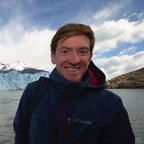# Image restoration for nanoparticle tracking

First published:

Last Edited:

Number of edits:

In video microsopy there are different effects that can distort the acquired images. For example, there are geometrical distortions generated by aberrations in the optics, there can be non-uniform contrast given by non-uniform illumination and optical transmission, and of course there is also noise. To perform nanoparticle tracking experiments, it is important to have a consistent method of dealing with background and noise that allows to normalize all the images in a sequence to the same characteristics.

While long-wavelength contrast variations waste the digital imaging system’s dynamic range, noise actually destroys information ()

## Long-wavelength background modulation

In the case of uneven illumination, the background signal will be modulated with a wavelength longer than the features that one wants to observe. Therefore we can model the background using a boxcar average in two dimensions ():

$$A_w(x, y) = \frac{1}{(2w+1)^2}\sum_{i, j = -w}^{w} A(x+i, y+j)$$

In the equation above, A is the image itself, while w is an integer number larger than the size of the particles but typically smaller than the interparticle distance. This definition of background is, perhaps, the simplest, and it neglects that videos also have temporal information.

## Noise in the images

Noise is intrinsic to the sensor in the cameras, and we can assume it is completely uncorrelated, this means that the correlation length is 1-pixel. If we would perform a 2D-gaussian filter of the image, we could remove the noise by washing out high frequencies while still retaining the information of the longer-range features, such as particles. The convolution of the image A with a Gaussian can be calculated as:

$$A_{\lambda_n}(x, y) = \frac{1}{B}\sum_{i, j=-w}^{w}A(x+i,y+j)\textrm{exp}\left(-\frac{i^2+j^2}{4\lambda_n^2}\right)$$

And:

$$B = \left[\sum_{i=-w}^{w}\exp\left(-\frac{i^2}{4\lambda_n^2}\right)\right]^2$$

## Defining a convolution Kernel

Using both the information for the background and the noise, we can define a single convolution kernel:

$$K(i,j) = \frac{1}{K_0}\left[\frac{1}{B}\exp\left(-\frac{i^2+j^2}{4\lambda_n^2}\right)-\frac{1}{(2w+1)^2}\right]$$

Where

$$K_0$$
is defined as:

$$K_0 = \frac{1}{B}\left[\sum_{i=-w}^{w}\exp\left(-\frac{i^2}{2\lambda_n^2}\right)\right]^2 - \frac{B}{(2w+1)^2}$$

note that

$$\lambda_n$$
is typically set to
$$1$$
,the correlation length of the noise in the images.

Of course, the methods above do not take into account problems that arise from saturation, nor explicitly defines what happens in the edges of the image. Most likely the authors are cropping the final image in order not to have to deal with the problems of a 2w sliding window.